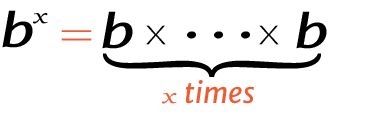# 8 raise to the power of 5

Place the base and exponent in the power calculator to get the right answer for value of 5^8, 58 (5 power 8), or 8 raised to the power of 5.

## How to calculate 5 raise to the power of 8?

This is how you can solve (5) 8 manually.

Solution:

Step 1: Simplify the data.

Base
ExponentStep 2: Multiply the 5 to itself 8 times.

Using this exponents calculator could be much easier to figure out an exponential expression.